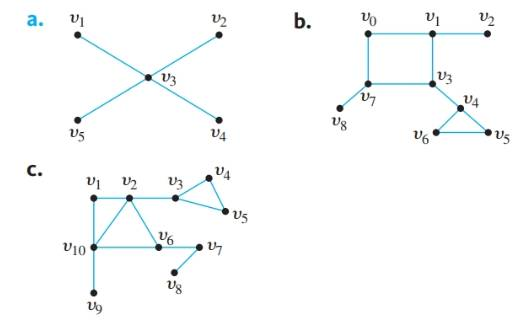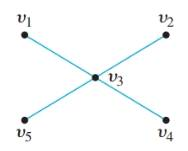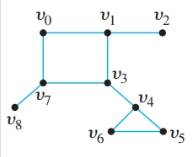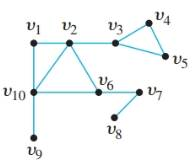Chapter 10.1, Problem 6ES### Discrete Mathematics With Applicat...

5th Edition
EPP + 1 other
ISBN: 9781337694193

#### Solutions

Chapter
Section### Discrete Mathematics With Applicat...

5th Edition
EPP + 1 other
ISBN: 9781337694193
Textbook Problem
1 views

# An edge whose removal disconnects the graph of which it is a part is called a bridge. Find all bridges for each of the graphs at the top of the next page.To determine

(a)

Find all bridges for each of following graph.Explanation

Given information:

An edge whose removal disconnects the graph of which it is a part is called a bridge.

To determine

(b)

Find all bridges for each of following graph.To determine

(c)

Find all bridges for each of following graph.### Still sussing out bartleby?

Check out a sample textbook solution.

See a sample solution

#### The Solution to Your Study Problems

Bartleby provides explanations to thousands of textbook problems written by our experts, many with advanced degrees!

Get Started